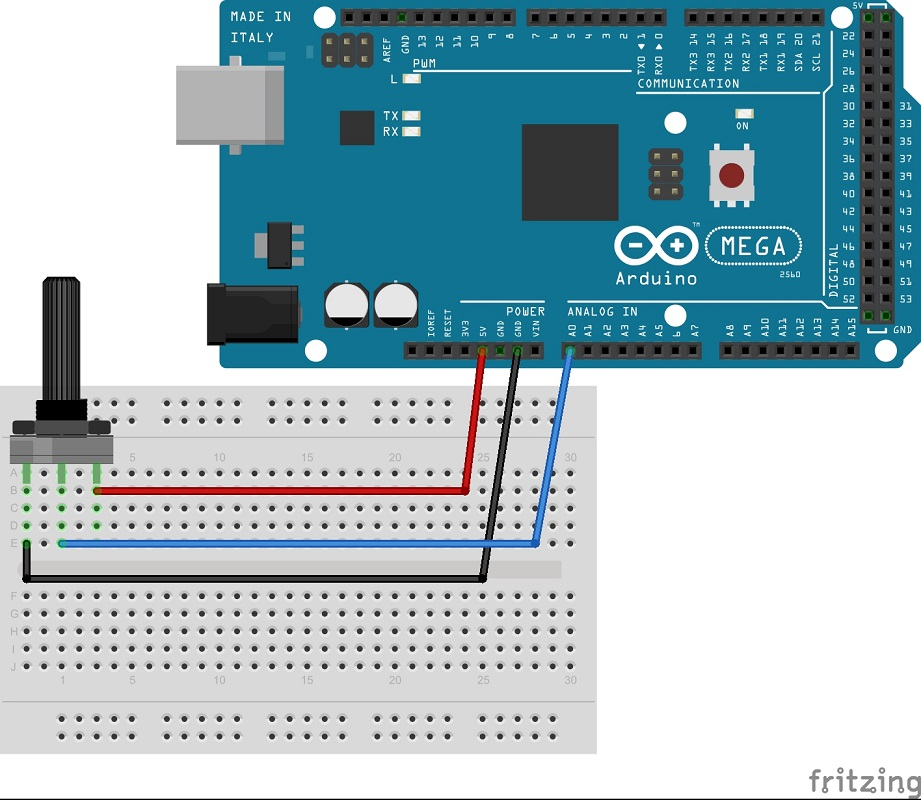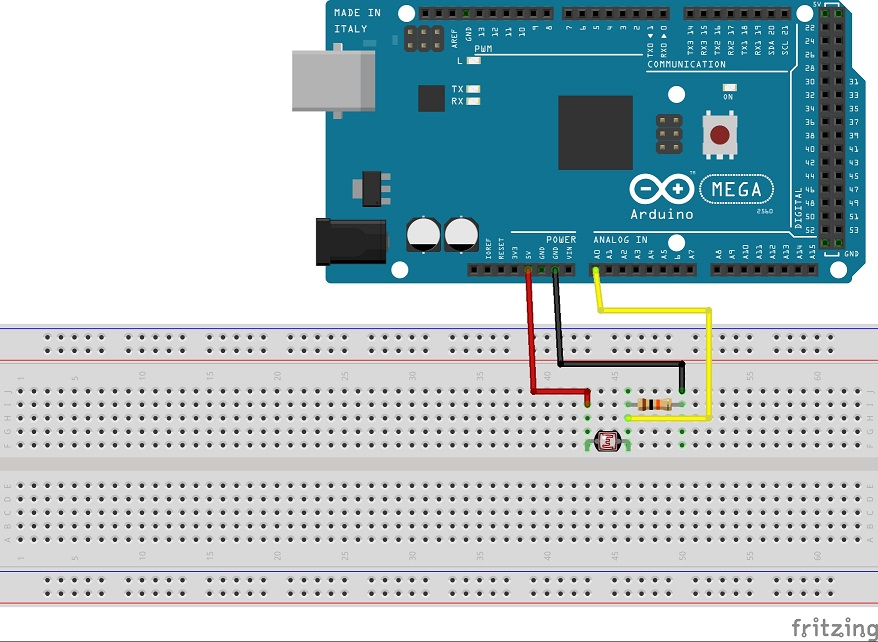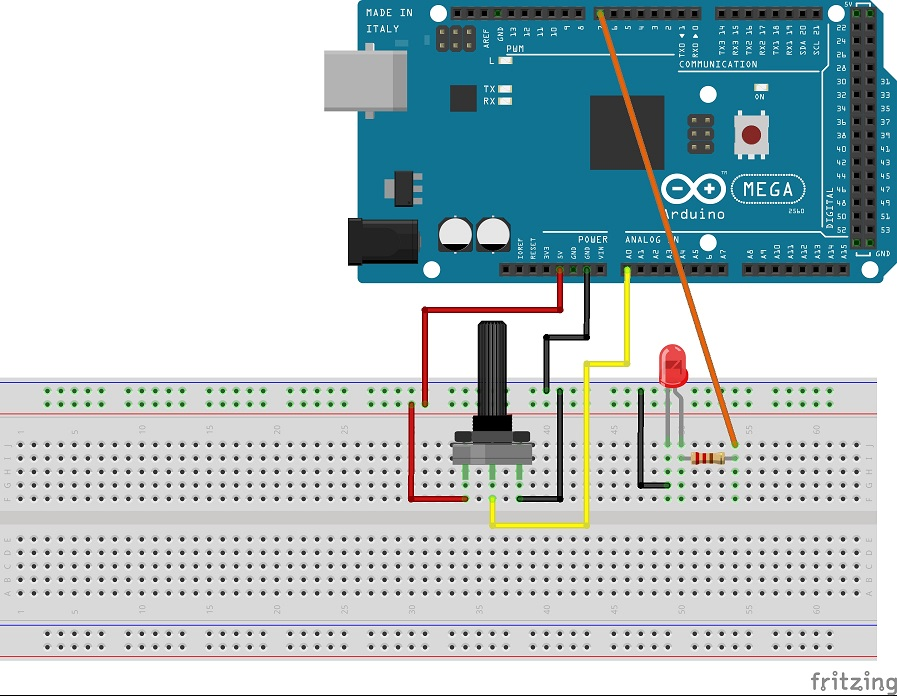Welcome to the third Arduino Tutorial from our Arduino Tutorial Series. In this tutorial we will learn how the Arduino Analog Inputs pins work and make few examples using a potentiometer and a photocell.

This is a Step by Step Video Tutorial which is easy to be followed. Also, below the video you can find what Parts do we need for this tutorial and the Source Codes of the Examples in the video.

Components needed for this Arduino Tutorial

Disclosure: These are affiliate links. As an Amazon Associate I earn from qualifying purchases.

### Circuit schematic of the first example. Using the potentiometer value as analog input### Circuit schematic of the second example. Using the photocell as a voltage divider and it’s variable value as analog input### Source Code of the first and the second example

```void setup() {
Serial.begin(9600);
}

void loop() {
Serial.println(sensorValue);```

### Circuit schematic of the third example. Using the potentiometer to control the LED brightness via PWM### Source Code of the third example

```int led = 7;

void setup() {
Serial.begin(9600);
pinMode(led, OUTPUT);
}

void loop() {
int newValeu = map(sensorValue, 0, 1023, 0, 255);
analogWrite(led, newValeu);
}```

### 10 Responses

1.Mr_Funkyman

good job, thank you!

2.Liam

Hi there
Would you be able to post code to control 2 stepper motors with a potentiometer, I have tried myself by cant seem to work it out.
Thanks

•Dejan Nedelkovski

3.stark

how can I control 2 motors with two potentiometers?

I tried to duplicate the code twice and changing the names so that it can read from A0 and A1 but didn’t work

•Dejan Nedelkovski

Sure, you can do that. You can’t duplicate the entire code twice, you need to duplicate and rename the motor and potentiometer definitions.

4.Alex

Hi Dejan,
Awesome tutorials, really great job! Many thanks!
It would be even better if you could mention values (parameters) of the passive components that you use, i.e. resistors, capacitors, sensors, etc. Newbies like me may well understand the logic and schematics but be unfamiliar with the usual parameters of components typically used with Arduino boards and most common breakout boards and controllers…
Many thanks anyway )
Alex

•Dejan

Thank you! I will take this into consideration.

5.•